# Java Mortgage Calculator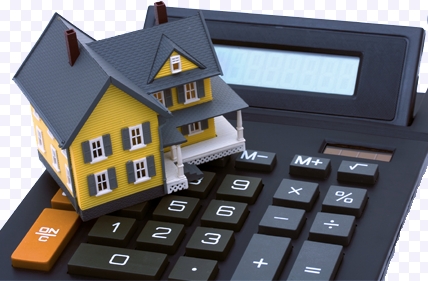Let’s have Java do the math for us!

## First Off, Mortgage Calculator?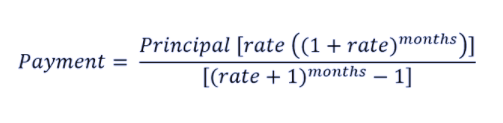Figure 1.0 The mortgage rate per month formula

## Initial Set Up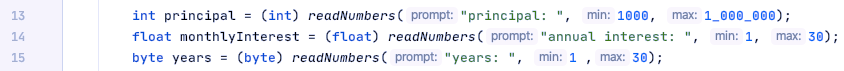Figure 1.1 These are the edge cases to make our mortgage calculator more realistic. The readNumbers is a method that reduces the amount of while loops used (left gif).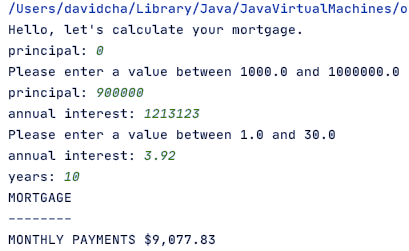Figure 1.2 This is our end result. The methods we create will take 3 inputs and calculate the monthly payments and will show the recurring payments which we see on the left.

## Let’s Start Building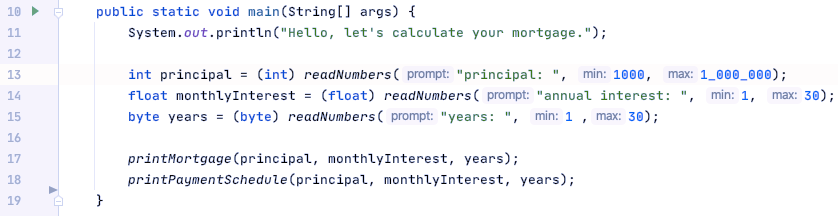Figure 1.3 Looks very beautiful. It only contains 6 lines of code.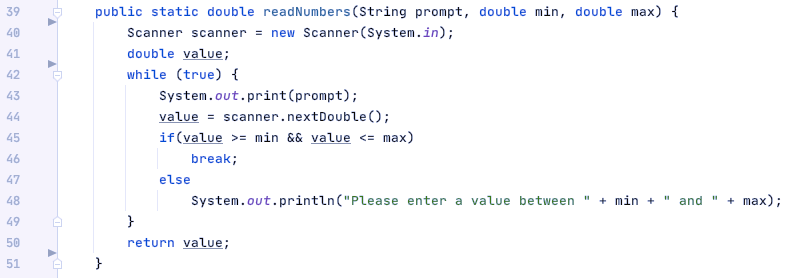Figure 1.4 We compare the values to the min and max with a while loop. The while loop will never end and will keep asking to input a value between the min and the max.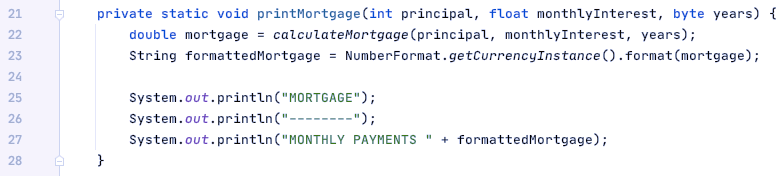Figure 1.5 This method is going to calculate the mortgage and it is going to take the mortgage value and abstracting the number values by changing it into a currency value.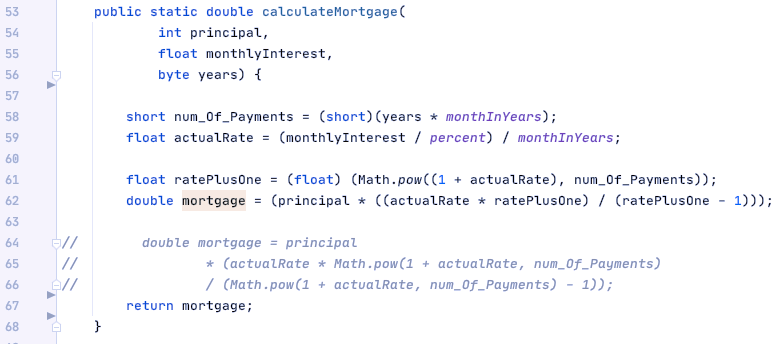Figure 1.6 Deconstructed formula of Figure 1.0. The variable monthInYears and percent are global variables I used that are outside the method scoop. I did it this way so that I don’t have to excessively type the same values.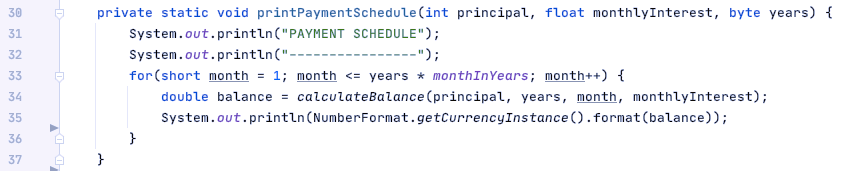Figure 1.7 It requires the same inputs from readNumbers and we are iterating the amount of month it takes with a for-loop to calculate the remaining balance.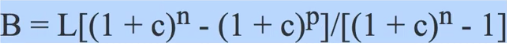Figure 1.8 The formula for the remaining balance from the mortgage.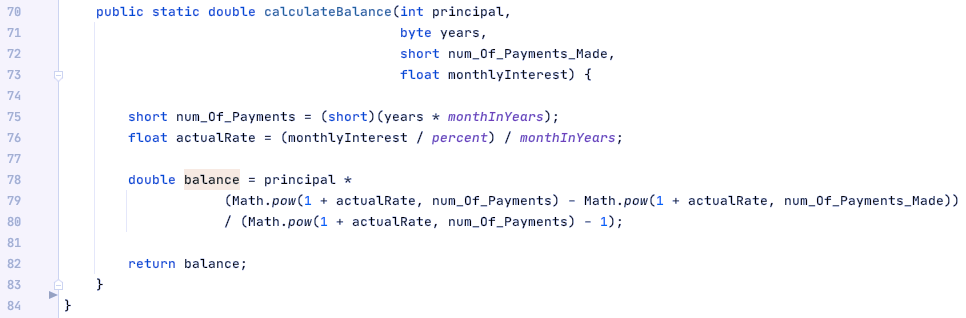Figure 1.9 Follows the same structure as figure 1.6.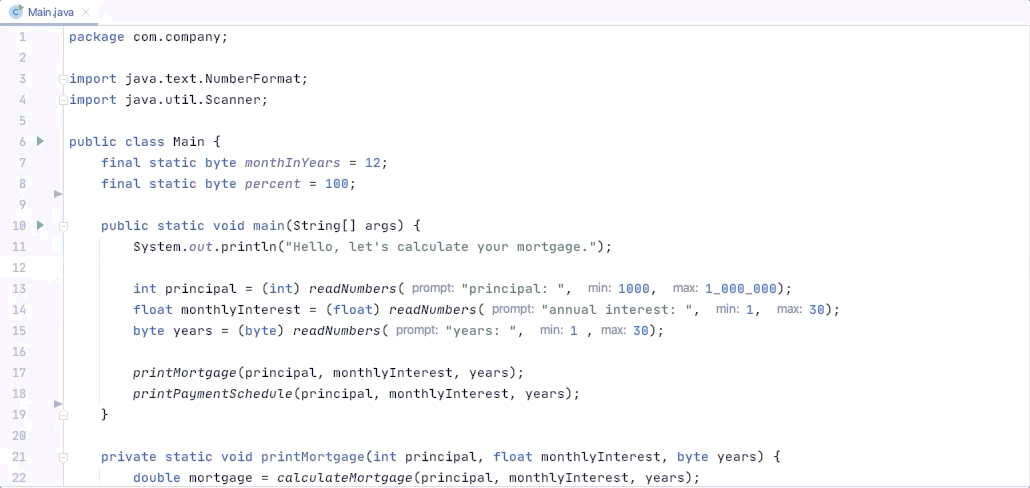More than 70 lines of code on a single method (left). The best practice is having each method contain about 10 to 20 lines of code (right). Sorry if you can’t read it, I used Giphy Capture to show the visual difference between using one methods and multiple methods for our calculator. I hope that you can see the visual difference and see how the code structure on the right looks more beautiful compared to the left.

--

--## David Cha

Interested in the structure of codes and chasing the Dopamine rush as I start to understand their structures.